# Bihar Board 12th Physics Important Questions Long Answer Type Part 2

BSEB Bihar Board 12th Physics Important Questions Long Answer Type Part 2 are the best resource for students which helps in revision.

Question 1.
Obtain an expression for refraction of light by double spherical surface or thin lens.
Or, Prove that $$\frac{1}{f}=(\mu=1)\left(\frac{1}{R_{1}}-\frac{1}{R_{2}}\right)$$
Let AB and CD are the two spherical surface. Its radius of curvature are R1 and R2. Refractive index of medium is μ. So these two acts as a thin lens having the same principle axis.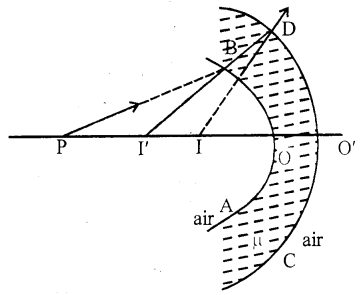Let P be and object on the principal axis. PB ray is incident on AB surface. After refraction it seems coming out form I’.
Hence, for AB surface
Distance of Object = OP = -u
Distance of image = OI’ = -ν’
By formula of single spherical surface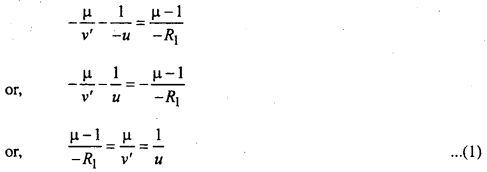I’ acts as an object for CD surface Image form at I by this surface.
∴ Distance of image = O’I’ = OI’ = -v’
[OO’ is supposed to be very near so O’I’ = OI’]
Distance of image = O’I = OI = -ν
from single spherical formulaWhen spherical surface AB and CD are combined, it acts as a thin lens. According to lens properties, if u = ∞ then ν = f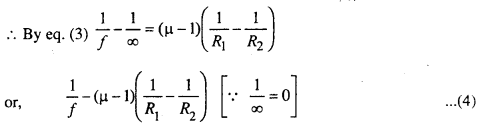Eq, (4) gives relation between f, ν and R. It is valid for both the concave and convex surfaces.
But for convex lens, R1 = +ve and R2 = -ve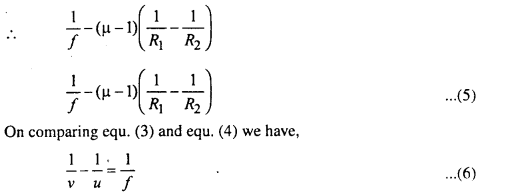equ. (6) gives relation between u, ν and f.

Question 2.
Obtain the value of equivalent focal length of two lenses placed at some distance.
Equivalent focal length of two lens placed at some distance: Let L1 and L2 be the two thin lenses co-axially at a distance d apart. Their focal length are f1 and f2.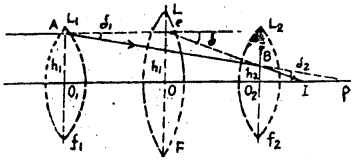Let the object is at infinite. Rays incident on point A parallel to the principal axis at height h1 from the opitical centre O1 of L1.

After refraction, it moves to point p on the principal axis.
∴ O1P = Distance of image = focal length (f1)

But the ray after refraction from the lens L1 becomes incident at the point B of lens L2 and finally after refraction from L2 converges at I. Hence for lens L2 the point P is the virtual object and I the real image.

Now is place of the two lenses a single lens L of focal length P kept in such a way that a ray from an object at infinity is incident at C and after refraction converges to I.

∴ OI = distance of image = focal length (P)
The magnification of the image at I is the same in both cases. Hence the lens L is equivalent to the lenses L1 and L2.
Let the incident ray be deviated by δ1 after refraction.Now in ΔACB
Exterior angle = sum of interior angles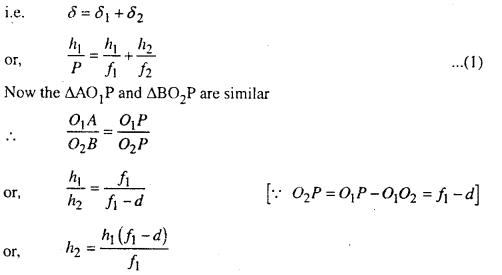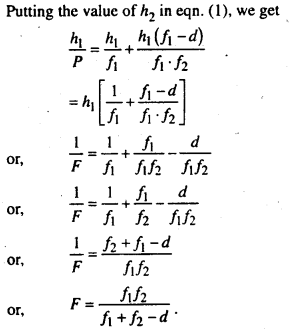Question 3.
Explain Huygen’s Principle of secondary wavelets and on the basis of this principle establish the law of reflection or refraction.
Huygen’s Principle’: This principle is only a geometrical construction which is used to determine the new position of the wavefront at a later time from its given positive at any time.

According to him (i) each points on the given or primary wavefront acts as a new source of secondary source secondary wavlets sending the disturbance in all directions as a similar manner as the orginal source.

(ii) The new position of the wavefront at any instant (called secondary waveforms) is the envelope of the secondary wavelets as that instant.

Explanation: Let the light point source sends wave in all direction an after time ‘t’ all particles on the surface AB will vibrate in the same phase. In this way AB is realy a part of sphere of radius ct and entre ‘s’ where c is velocity of light. AB is called primary wave front. If the distance is small wavefront will be spherical but when distance is large the portion of wavefront will be consided as plane.According to Huygen, all points of primary wavefront become a source of secondary wavelets. These wavelets trave with same velocity in all direction as primary. At the end of time interval (t + Δt) the enveolve of secondary wavelets of radius (c Δ t) becomes the new wavefront (A’B’) or secondary wavefront.

Huygen assumed that the portion of secondary wavelets, which lies on the backward secondary wavefront does not exist at all. He could not be explained it was why? However latter on Vaigt and Kirchhoff mathematically proved that the contribution of a wavelet in any direction making angle θ which the normal to the wave let is proportional to $$\frac{1}{2}$$(1 + cosθ). Obviously, for the portion of the wavelet, which lies on the just back of the secondary wavefront θ is equal to 180° and likewise the factor $$\frac{1}{2}$$(1 + cosθ) is equal to zero. Therefore, the forward wavefront A’B’ is the new position of the wave front at time t + Δt.

(a) Law of reflection on wave theory:
Let plane wave front ABC falls on reflecting surface AE making ∠i of incidence. During the time ‘t’ when the point C of incident wave front reaches of point E of the mirror, the secondary wavelet produced by the point A of primary wavefront becomes a sphere of radius AG.
i.e. AG = CE = CE where C is vilocity of light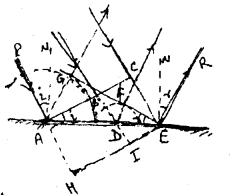Let EG be a tangential plane. Now if two waves produced from any point B of the primary wavefront just touches the plane EG at the end of time ‘t’, them it must be reflected wavefront.

Clearly during the times ‘t’ the point B of primary wavefront touches the point D of mirror and reached at point-I in the absence of mirror. Now DF ⊥ EG drawn.
∴ ∆AEG ≃ ∆OEF
∴ $$\frac{A G}{D F}=\frac{A E}{D E}$$ …………(1)
In the absence of mirror, ABC will be in the position HIE at the end of time t.
Clearly, AH = CE = AG = Ct and from ∆AHE ≃ ∆DIEThus at and of time ‘t’, the point B of incident wavefront will be at F in presence of mirror which is at I in the absence or mirror. Therefore, EG is reflected wavefront.This is second law of reflection. Clearly the incident ray. normal to incident point and reflected ray all lie in the plane of paper i.e. is the same plane. This is the first law of reflection.

(b) Law of refraction:
Let plane wavefront ABC incidents on the surface AE making an incidence angle I separating the two media. 1 and 2 in which C1 & C2 are velocities of light respectively.

In the time (t) when the point C of the incident wave-front reaches to point E of the separating surface, the secondary wavelet produced by point A becomes a sphere of radius AG in the second medium where AG – C1t and CE – C1t. Let us draw a trangular at surface EG. The plane EG will become refracted wavefront, if we show that the wavelet produced from any point B of the incident wavefront, just touches the plane EG at the end of time ‘t’.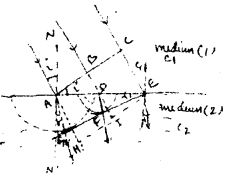The wave let produced from part-B touches at D of the inter t. from D, DE ⊥ EG is drown.
Then from similar ∆AGE & ∆DFE.
$$\frac{A G}{D F}=\frac{A E}{D E}$$ ………..(1)
In the absenge of medium 2. at the end of time ‘t’ the position of ABC is HIE and AH = C1t
∴ from similar ∆AHE & ∆DIE⇒ the time taken by wave to reach from D to F in medium 2 is equal to the time tank by wave to reach from D to 1 in the medium I. Thus EG will be refracted wave front.
Now ∠GEA – ∠r = angle of refraction.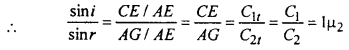when 1µ2 is refractive index of medium 2 with respect to medium 1. This snell’s law.
Clear from fig. the incident ray, normal to incident point and refracted ray all lies in the plane of paper i.e. all are coplanar. This the first law of refraction.

Question 4.
Describe with theory for measurement of wavelength of Sight by Fresnel biprism, or, Measurement of width of fringe.
Fresnel biprism: Fresnel biprism is made up of two acute angled prisms having their bases in contact with each other. The acute angle is of approximately one minute. In actual practice to prism is grounded from one single optical plate. Fresnel’s biprism is used for obtaining two coherent sources.

Formation of Fringes: S is a light source. The prism P reflects the light the source and forms two virtual images S1 and S2. These two images reserve as the two coherent sources of light and produce interference pattern in the region PMDENP which are obtained on a screen AB. On the screen DOE is the region where the two light beams from S1 and S2 overlap and the darkness or brightness at any point in this region will depend upon the path difference only of the two light screen.Theory: Let the wavelength of the monochomatic light emitted by S1 and S2 by λ. A screen AB is placed at a distance D from the two sources which are suppose a part by a distance from S1 and S2 so there will be no path difference (so to say no phase difference) hence at O there will be a central bright fringe. Above or below the point O there will be alternately dark bright fringe due to difference in the path difference.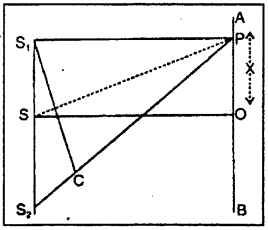Let OP = x join S1 P1 S2 P and SP and a perpendicular S1C is drawn on S2P. As the point P is very near the point O, S1 C may be considered to be perpendicular to SP. As the two angles S1, CS2 and POS are right angles, hence the two triangles S1 S2 C and SPO are similar.In order to the point P be position of bright fringe the path difference CS2 should be an even multiple of λ/2.In order to the point P to be the position of a dark fringe the path difference CS2 should be an odd multiple of λ/2.
So, CS2 = (2n + 1) λ/2, where n = 0, 1, 2, 3 ….The distance between two consecutive bright fringes β is given bySimilarly the distance between two consecutive dark fringes i.e. β’ is given by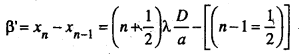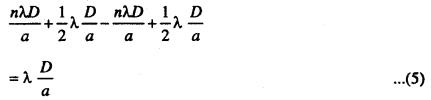By comparing the equation (4) and (5). We find that b = b’ both the bright and dark fringes are equally spaced.
So the fringe width β = $$\frac{\lambda D}{a}$$
or, λ = $$\frac{\beta a}{D}$$ ………..(6)
Experiment details The experiment for determining the wavelength of a monochromatic light using formula λ = $$\frac{\beta a}{D}$$ is performed on an opticle bench.

In the stands connected with the optical bench the bi-prism slit and an eye-piece is fixed and the slit is illuminated by a monochromatic light source.

The cross wire of the eye-piece is made to coincide with a bright firing and the reading on the micrometer scale of the eye-piece is noted. The micrometer screen is shifted side ways (either to left, to right) and is again made to coincide with another bright fringe and the number of fringes the pass through the field of view is counted. The reading on the micrometer screw is again noted. Hence the distance between two consecutive bright firings is calculated. Then the distance between the slit and the eye-piece D is determined.

In order to determined the distance between the two virtual images of the sources a convex lens is placed on another stand on the optical bend between the bi-prism and the eye-piece without disturbing their position. The position of the convex lens is so adjusted that a sharp image of S1 and S2 is obtained in the eye-piece. The distance between the two images determined by using the micrometer scale. Let it be d1 the lens is then shifted to another position till a sharp images of S1 and S2 are again obtained and the distance between them is again measured. Let be d2.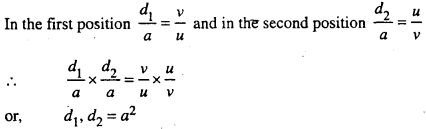or, a = $$\sqrt{d_{1} d_{2}}$$
Thus knowing α, β and D the wavelength λ of the monochromatic light can be calculated.

Question 5.
Find expression for instantaneous current in LCR circuit Also frnd expression for power in LCR circuit
LCR Circuit: In fig (1), an inductor coil (L) capacitor (C) and resistor (R) are connected with an ac source of emf e = e0 sin wt. in series. The instantaneous current of ac circuit is given by i = i0 sin (ωt + Φ) ………….(1)
Where i0 = $$\frac{e_{0}}{z}$$ = peak current and z = $$\sqrt{R^{2}+x^{2}}$$ = impedance of the circuit and Φ is called phase constt. of the circuit.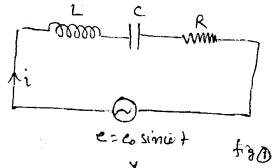For finding the value of impedence Z and phase Φ of the cirucit, we draw phasor diagram or vector diagram. For this purpuse, vectores along X and Y axes are drawn taking the magnitude equal to resistance R along X- axis and inductive reactance XL = ωL along +ve y-axis and capacitive reactance Xc = $$\frac{1}{\omega C}$$ along -ve y-axis. The resultant of resistance R and reactance X = XL – XC gives the impedence Z = $$\sqrt{R^{2}+X^{2}}$$clearly from eqn. (2) the value of impedence depends upon reactance and depends upon the the freq. of the source also. For a particular freq. = wr, XL = XC and net reactance become minimum so that peak current = $$\frac{c_{0}}{z}=\frac{c_{0}}{R}$$ will be maximum. In such a case, the circuit is called resonant circuit and the w = wr is called resonant freq. The phase constant is given by
tanΦ = $$\frac{X}{R}=\frac{X_{L}-X_{C}}{R}$$
Thus from eqn. (1) the instantaneous current i is determined.
The average power of a.c. is given by
Pav = erms irms cosΦ
and power factsFor resonant circuit power factor cosΦ = $$\frac{R}{R}$$ = 1 (max)
Therefore power consumption in resonant circuit is maximum.

Question 6.
What is mean by radio activity? Describe the nature an properties of rays emitted by radio active substance.
Or, Describe the properties of α, β, γ rays.
Radio activity: Radio activity was discovered by Becquerel in 1898. It is spontaneous phenomenon exhibited by heavy element of atomic weight greater than about 206. It was later on shown by rutherford that the radiations emitted form radioactive substance are of three types. The radioactive substance is taken in a thick walled lead chamber provided with a small opening at the top. When a magnetic field is applied at right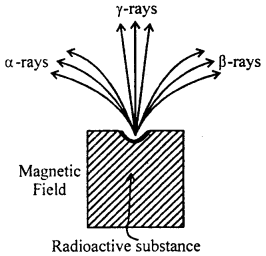angles to the direction of the rays, some of the rays are found to come out undeflected and other ray are deflected either towards the right or the left of the undeflected component. These undeflected rays are called y-rays and the other rays are found to carry either positive or negative charges as is concluded. From their direction of deflection. There are respectively named as α-rasy and β-rays. Uranium is radioactive substance. In addition on this there are other substance also such as thorium, Radium, polodium etc. which are also radioactive.

Nature and properties of rays emitted by radioactive substances:
α-rays:

• α-rays are positively charged particles and are deflected by a magnetic as well as an electric field.
• α-rays have been identified as doubly ionised helium atoms.
• These rays can ionise gases through which they pass.
• The velocity of α-particles are smaller compared to that of β-particles but is may be as high a 1.5 × 107 m/s.
• α-rays can be stopped by a very thin sheet of metal.
• When α-particles fall on a zinc sulphide screen, scintillations are produced.

β-rays:

• β-rays are negatively charged particle. Actually they are steam of electrons.
• β-rays are also deflected in magnetic field but their deflection is in opposite direction to that of α-rays.
• The velocity of β-rays are very high, but as their masses are very small, their kinetic energy is small.
• They have high penetrating power than α-rays.
• β-rays do not produce sufficient ionisation in gases.

γ-rays:

• γ-rays differ greatly form the α and β-rays. They are neither deflected by an electric field nor by a magnetic field.
• γ-rays are not charged particles.
• These rays have much greater penetrating power than the β-rays.
• γ-rays have very high penetrating power and can penetrate even a sheet of iron of thickness 0.3 meter.
• They have very feeble ionising power.
• γ-rays can effect photographic plate and can also produce flurscence on certain substance.
• There rays are used for the treatment of cancer.
• The wavelength of γ-rays are as low as 0.007Å i.e. less than the wavelength of x-rays.

Question 7.
What do you understand by radio active disintegration and radio active constant? Explain the term half life period and mean or average life.
Radioactive disintegration: Radio active element spontaneously disintegrate giving out α, β and γ radiation. In this way new atoms are formed. Uranium on disintegration gives rise to a new substance lead.
The above facts can be explained on the following law by Rutherford.

• The disintegration of radio active element is purely a random process and its is merely a matter of chance, which nucleus will suffer disintegration or decay first.
• Atoms of every radioactive element are constantly breaking into fresh radioactive products with emission of α, β and γ rays.
• The rate of decay is directly proportional to the quantity of material actually present at that instant and this is independent of any physical or chemical state.

Let at any instant “t” the number of atoms in a radioactive substance is N. After d time, the number of atoms disintegrated is dN.

Hence from third law,
$$\frac{d N}{d t} \propto N$$
= -λN …………(1)

Where λ is the decay constant or disintegration constant, (-ve) sign shows that with time the rate of disintegrating the atoms reduced.
or, $$\frac{d N}{N}$$ = -λdt
On integration,
loge N = -M + C ………….(2)
Where C is constant of integration
Let n the instant i = 0, N = N0 then
loge N0 = i + C = C
Putting the value of C in eq. (2)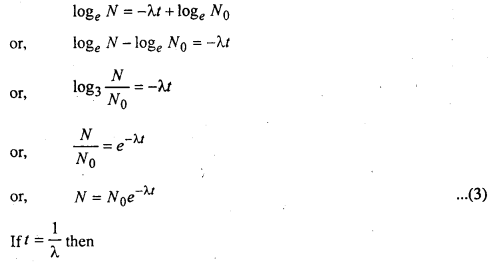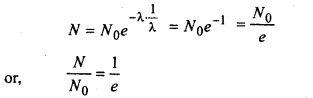Hence the radioactive constant is also defined as the receprocal of the time during which the number of atoms of a radioactive substance falls to $$\frac{1}{e}$$ of its original value.

Half life- The half life of a radioactive substance is the time in which the number of radioactive atoms of the substance is reduced to half of its original value.Mean or Average life:
Mean life is defined as the ratio of the sum of the lives of all the atoms and total number of atoms.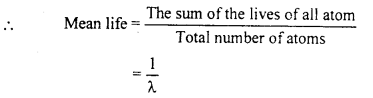Hence mean life of a radioactive substance is the reciprocal of its decay constant.

Question 8.
On the basis of energy b and distinguish between conductor, insulator and semiconductor.
Metals are good conductors of electricity, insulators do not conduct eletricity, while the conductivity of semiconductors lies in between those of good conductors and insulators.

Distinction between conductors, insulators & semiconductors on the basis of band theory of solid:
A solid is large collection of atoms. The energy levels of an atom get modified due to the presence of other surrounding atoms and the energy levels in die outer most shells of all the atoms form valance band and the conductor band separated by a forbidden energy gap.

The energy band formed by a series of energy levels containing valence electrons is called valance band. At OK, the electrons start filling the energy level in valance band starting from the lowest one. The highest energy level. Which an electron can occupy in the valance hand at OK is called fermi leval. The lowest unfilled energy band formed just above the valance band is called conduction band.

At zero kelvin the Fermi level as wells as all the lower energy levels are completely occupied by the electrons. As temp, rises, the electrons absorb energy and get excited. The excited electrons jump to the higher energy levels. These electrons in the higher energy levels are comparatively at-larger distance from the nucleus and are move free as compared to the electrons in the lower energy level. Depending upon the energy gap (E ) between valance band and conductors. The solids be have as conductors, insulators and semiconductor as explained below-

(a) Conductors (Metals): The band structure in solid have Possibilities:
(i) The valance band may be completely filled and the conduction band partially filled with an extremely small energy gap between them, fig(i)
For example: Sodium like metals have only one electrons in outer most orbit. Hence its conduction band is partially field.(ii) The valance band is completly filled and conduction is empty but the two overlap each other fig. (2) There is no energy gap between them. Zinc like metals have two electrons (which is completely filled) in oularmos orbit hence its conduction band is empty but valance band (completely filled) overlap on the CB.In Both the situation, we have only one energy band which is partially filled and therefore, on applying even a small electric field, the metals conduct electricity i.e. they are good conductor of electricity.

(b) Insulators: ln insulators the forbidden energy gap is quite large fig. (3) for example, the forbidden energy gas for diamond is σeV. Which mean that a minimum of σeV energy is required to make the electron jump from the completely filled valance b and to the conduction band.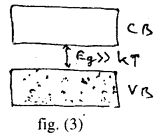(c) Semi-conductors: The energy and structure of semiconductors is similar to the insulators but in their case, the size of the forbidden energy gap is much smaller than that for the insulators fig (4). For example the forbidden bond for Si is 1.1 eV the electronic structure of Si is similar to that of diamand, but due to smeller width of forbidden energy gap, the electrons in the valance band find it comparatively easier to shift to the conduction band. So conductivity of the silicon is inbetween the conductor and insulators and it is termed as semiconductors.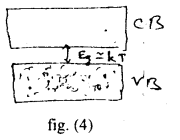Question 9.
(i) How p-n is used as Diode?
(ii) Establish relation between mass and energy.
(i) p-n in as a Diode: We imagine a semi conductor. One part of which is p-type and other one is n-type. AB is the dividor.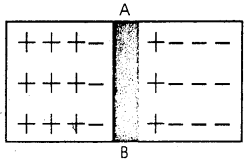This is called “p-n junction”, n-type contains more electron. So it moves to the pther part of fill up hole. On the other hand p-type contains positive holes. So it moves to n-type for combination with electron. Due to this reason, positive charges is reduced in the p-type and electron in the n-type. A layer of changs forms at the jn, and causes a potential difference that tends to stop electron and +ve holes to cross this barries. This potential is called Brarrier potential.

If p-type of p-n junction is joined to +ve pole of cell and n-type to -ve pole, then this connection is called “forward bias connection.”If -ve of cell is attached to p-type and +ve of cell is attached to n-type then this is called “Reversal Bias connection.” On increasing current by increasing potential of Bias covalent bond beaks-near the junction. Due to this reason electron and +ve hole produces. This is called breakdown point.

AC can be transferred into D.C. by p-n jn. on supplying A.C. in the circuit in half period it will work as a “forward bias connection” so potential difference is obtained as cross R. In second half the connection work as “Reverse bias” which give almost zero current. So we get neglible potential difference a cross R so p-n jn. work as “Half wave reactifier.”

For full wave rectifier, two p-n jn is taken in the circuit.

(ii) Mass-energy equivalence:
Before Einstein, it is assumed that the mass of a body is considered to be constant not depending upon the velocity of body. But the theory of relativity given by Einstein says that the velocity of the body cause change in mass of body. Mass and energy are equivalent energy of a body increases with loss of mass.

Einstein proposed the following formula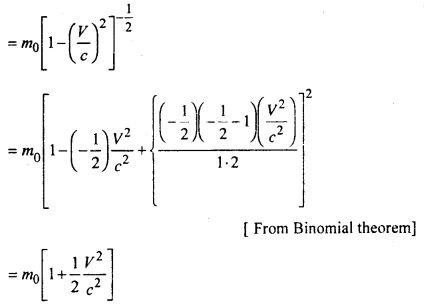Where $$\frac{1}{2}$$m0ν2 = Ek (kinetic energy of a body and (m – m0) = δm (change in mass)
In general E = mc2

Hence kinetic energy of a body is equal to mc2. If the velocity of the body in equal to speed of light then the mass of body becomes infinite.
This is called einstein is mass-energy equation or mass-energy equivalence.

Question 10.
What is logic gate? Discuss its different kinds.
Manipulation of Binary notations is done by logic circuit called gates. So logic gate or logic circuit is the circuit which is used us switch and gives the relation between input and output.
Closed switch is denoted by 1 (one) and open switch is denoted by 0 (zero)

Logic gates are of following types:
1. OR Gate: One output is produced by two or more inputs.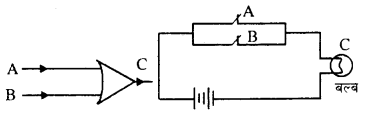A and B are two switch attached in parallel. Bulb C and cell are also attached. A and B acts as input and C works as output C does not lights on it A and B both are open. C lights it A or B is closed. The truth table is shown. Boolean equation of this gate can be given as

 Input Output A B C 0 0 0 0 1 1 1 0 1 1 1 1

C = A + B
i.e., C = A or B

2. AND Gate: Here also one output is produced by two or more inputs.A and B are two switches attached in series. Bulb C and cell are also attached, and acts as input and C acts as output C does not lights it A or B is open. C will be on only it. A and B both are closed. The truth table is shown Boolean equation. For this Gate is given as

 Input Output A B C 0 0 0 1 0 0 0 1 0 1 1 1

C = AB
i.e. C = A and B

3. NOT Gate: There is only one input and only one output in this Gate. It there is no input (A) the output will be found. If there is input given then output will be zero.A B 0 1 1 0

i.e. A = 0 then B = 1
A = 0 then B = 0

This gate is shown by drawing a circle at the output. This is called invert bubble. The Boolean equation is B = $$\bar{A}$$.

4. NOR Gate: The combination of Not gate and OR gate is called NOR gate which acts as NOT-OR circuit.

 Input Output A B C 0 0 1 0 1 1 1 0 1 1 1 0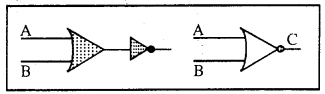The results are complementary to the OR Gate. Output C is one in both the inputs A and B are zero. Output is zero if any of A and B have some value. This is shown in truth table. Boolean equation can be given as
C = $$\overline{A+B}$$

5. NAND Gate: The combination of NOT gate and AND gate acts as NOT-AND circuit and called NAND Gate. This is shown by adding Not gate to the AND Gate.

 Input Output A B C 0 0 1 0 1 0 1 0 0 1 1 0Its action is just reverse or complimentary of AND gate. In this gate, if A and B are zero then output will be 1. But if A and B are 1 then output will be 0 (zero).
Its Boolean equation is $$\overline{A \cdot B}$$ = C.

Question 11.
What is a Laser? Give its basic principle. Describe Ruby laser. Give main uses of Laser. Name some important types of Laser.
Laser: The English word Laser is an abbreviation of “Light wave Amplification by stimulated Emission of radiation”. Towns and Schawlow in 1958 worked out the principle of Laser. A Laser Q is a device which produces a highly concentrated, monochromatic, coherent and unidirectional beam of light. The typical operating frequency of a laser is 1015 Hz invisible region.

A Laser is the modified form of maser which uses microwave instead of light. Maser is an abbreviation of “Microwave Amplification by stimulated Emission of Radiation.” Due to this reason laser is also called “Optical Maser.”

Basic principle: The principle of laser work on stimulated emission and self emission. In 1917 Einstein showed that stimulated atom comes in its lower energy state from higher energy state by emitting radiation in the following two ways-

• Without the effect of any external radiation, which is called self emission.
• In the presence of electro magnetic radiation of special frequency which is called stimulated emission.

At every temperature atoms of higher energy state and lower energy state are present in matter. Let there be Ni electrons per unit volume in energy state 1 and N2 electrons per unit volume in energy state 2. The energy level 2 is above the energy level 1. If energy of atom is transmitted from level 1 to level 2 then photons are absorbed and if it is transmitted from level 2 to level 1, then photons are emitted.After absorption of photons let us now see how the process of self emission or stimulated emission take place. To understand it we consider about energy level 2 of Ruby crystal of chromium.

The lives of E1 and E2 energy states of atoms decreases by 10-8 sec and life of M-energy level is 3 × 10-3 sec.

Energy state M is called meta stable state. Unstimulated chromium remains in ground state. By absorbing photons of 6600Å it comes to E1 energy state and also by absorbing photons of 4000Å it comes to energy state E2. After transition from E1 and E2 state it comes in meta stable state M.The energy produced from these transition is absorbed by crystal lattice. Thus during such transition emission of radiation does not take place.

When transition of atoms occurs from Metastable state M to ground state O then radiation of wave length 6900Å approx is emitted.

This is possible by stimulated emission. Due to this emission the power of radiation send in matter is amplified. To increase the power of radiation which is sent in matter, it is necessary that the population of atoms occupying lower energy state must increase. Thus it is necessary to increase the population of high energy atoms.

In forms of light amplification, the population at higher energy levels is more than that in lower energy levels.

In the atoms of which matter stimulated emission and population inversion takes place that active medium is placed between two plane mirror.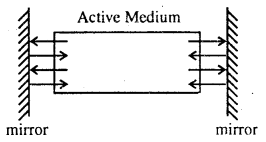Between these plane mirrors light beams begin to reflect continuously and during this process the strength of light amplifies successively. Such type of arrangement of mirror is called resonant cavity. To obtain light beam or the outside of the active medium one mirror of resonant cavity is made partially reflecting.

Types of Laser:

• Solid laser (Ruby laser)
• Liquid laser
• Gas laser – Helium-Neon lasers in the best known gas laser
• Semi-conductor laser
• Chemical laser
• Dye laser

Ruby laser: This is the first laser developed in 1960 and is a solid state laser. It consists of a pink ruby cylindrical rod whose ends are optically flat and parallel. One end is fully silvered and the other is only partially silvered. The rod is surrounded by a glass lube upon which is wounded a coiled flash lamp filled with xenon gas.

Working: A condenser charged to some volt is being discharged through flash lamp. The light gained by electric discharge of gas in flash lamp gives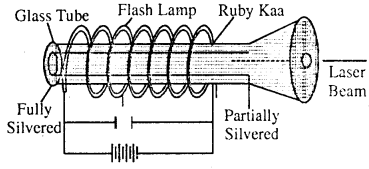stimulated emission from chromium atoms of the medium and the laser beam obtained by partially silvered end of the crystal, from it. we may get 10 kw peak power.

Uses of lasers: Laser has a number of practical application-

• Laser beam acts as a sharp knife and is used to perform bloodless surgery.
• Laser has been used for drilling holes in hard material like diamonds, painless drilling and welding of teeth etc.
• In high speed photography, it is used to obtain very sharp images of moving objects.
• In basic scientific research, laser has been used in exploring the molecular structures, nature of chemical reaction, Raman spectroscopy and accurate determination of velocity of light.
• It is also used for automatic control and guidance of rocket and satellite.
• Laser can also used to destory aeroplanes, missiles and tanks.

Question 12.
Explain Davisson and Germer’s Experiment to verify De-Broglie hypothesis.
Davisson and Germer’s experiment: Davisson and Germer experimentally demonstrated the diffraction of electrons.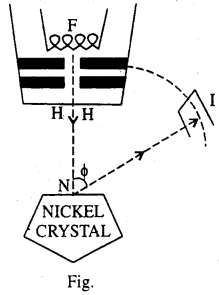The electrons are produced by heat ting a tungsten filament F and are accelerated through a known potential difference V by maintaining a stead potential difference between the filament F and a plate P.

The electrons are collimated by passing through narrow holes and finally emerge through fine hole H in the form of a very narrow beam. The whole arrangement is known as an Electron gun. The fine beam of electrons falls normally on the surface of a single. Nickel crystal N. This is prepared by baking the block of very small individual crystals of which the block is normally composed of form a large single crystal, all the atoms of which are arranged in a regular lattice. The electrons are scattered by the crystal in all directions and the intensity of the scattered beam in any direction is measured by allowing the beam to entre the ionisation chambers / set at appropriate angle and noting the deflection in a sensitive galvanometer connected to I.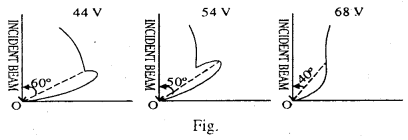The chamber can be rotated with the crystal as the center are the intensity of the scattered beam can be determined as a function of scattering angle Φ, the crystal being held in a fixed position throughout the experiment. The results of the experiment is presented in the form of a polar graph. In the polar graph, a line is drawn from O inclined at an angle Φ with Y-axis which drawn from O is taken proportional to the intensity of the diffraction electron beam. The end points of these lines drawn for diff erent value of f are jointed to get a smooth, continuous curve. This construction is known as the polar graph.

The observation were taken for values of voltage varying from 40 V to 68 V. The graph at first is fairly smooth but at a potential difference of 44 V a spur or hump appears on the curve at an and of a bout 60°. As the P.D. is increased the length of the spur increase and a sharp maxima is obtained at P.D. of 54V at an angle Φ = 50°. With further increase in P.D. the spur decreases in length and finally disappears at 68V at an angle of about 40°.

If we plot the intensity of electron beam against the angle Φ for a P.D. of 54V, we get a curve as shown in the Fig. This shows that electrons with kinetic energy 54eV suffer maximum scattering at Φ = 50°.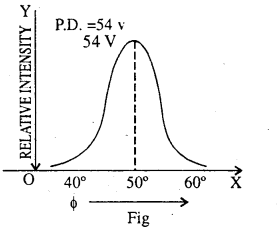Discussion: The de broglie wave length associated with the electron accelerated through a P.D. of 54 volts according to relation is given byThe wavelenth can also be calculated from the diffraction experiment as the lattice constant for a single crystal of Nickel.
For the first order maximum n = 1
λ = 1.65 A°
This result shows excellent agreement with calculated value of λ = 1.66A° from De-Broglie hypothesis.

Question 13.
What is nuclear reactor? Describe its construction and uses.
A nuclear reactor is a device in which enormous energy due to nuclear fission is produced by self controlled chain reaction.

Parts and function of nuclear reactor:

• Active Core: It consists of fissionable material called reactor fuel which can be $${ }_{92}^{235} \mathrm{U},{ }_{94}^{219} \mathrm{PU},{ }_{92}^{233} \mathrm{U}$$.The fuel is used in the form of rods.
• Moderators: The neutrons released by the fission of uranium have energies of the order of 2mev. By the use of moderators fast neutrons can be slowed down. In moderators used Heavy Water. Graphite of beryllium oxide etc.
• Coolants: In nuclear reactor generated Heat due to the fission and transfer it to steam by coolants. That steam rotates a turbine for the production of electric power. Coolants used are water, liquid and gases.
• Control Rods: To control chain reaction certain materials which absorb neutrons without becoming radioactive are used. Cadmium are used for control rods because of their ability to capture slow neutrons.
• Shield: Thick walls of cement and concrete are built around the reactor to stop the radiations coming out of reactor to avoid hazards of exposure.

Uses of Reactor:

• It is used for the generation of electric power.
• It produces plutonium which is used in atom bomb.
• It is used for the production of fast neutrons that are needed for nuclear bombardment.
• Radio isotopes of various elements are produced. The find extensive use in pure science, biology and medicine.

Question 14.
State the basic postulates of Rutherford and Bohr’s model of atom. Obtain the energy of orbital electron in hydrogen atom.
Rutherford Model of atom:

• All the positive charge and almost all the mass of the atom is concentrated in the central core, called nucleus.
• The nucleus is surrounding by electrons which revolve round the positively charged nucleus. The numbers of electrons in the outer part equals the number of protons in the nucleus so that atom is electrically neutral.
• Size of the nucleus is very small as compared to the size of the atom.

Bohr’s Model of atom: The basic postulates on which Bohr’s theory of hydrogen atom is based are as follows
(i) Bohr postulated that electron can revolve only in those orbits for which the orbital angular momentum of the electron is equal to an integral multiple of h.
i.e. angular momentum = η$$\frac{\mathrm{h}}{2 \pi}$$

(ii) An electron emits electromagnetic radiations only when it jumps from stationary orbit of high energy to the statiionary orbit of lower energy. The fraquency of the radiation thus emitted is given by:
E2 – E1 = hυ.

Energy of orbital electron in hydrogen atom:
The energy (E) of the electron in an orbit is the sum of potential and Kinetic energies. If ν is the velocity of the electron then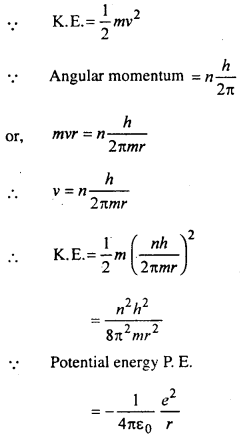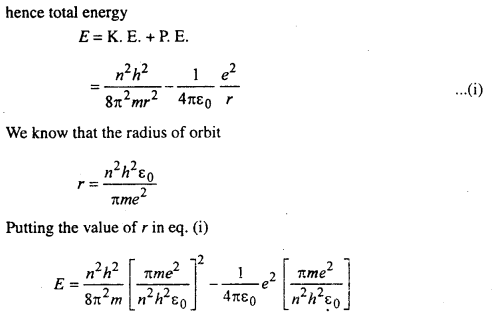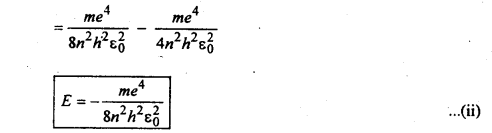eq. (ii) is the energy of the electron in the nth orbit.